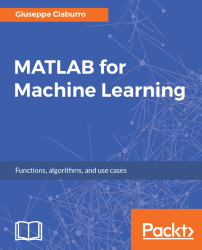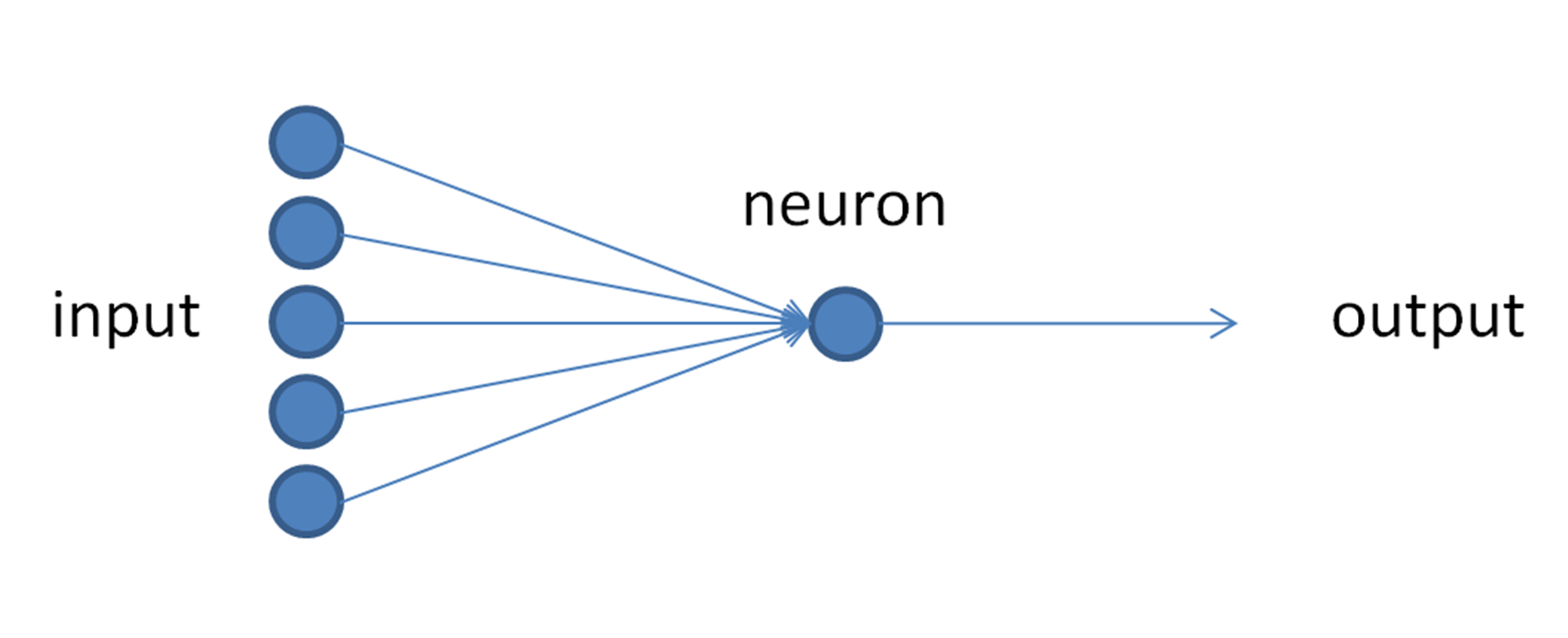•#### MATLAB for Machine Learning#### Overview of this book

MATLAB is the language of choice for many researchers and mathematics experts for machine learning. This book will help you build a foundation in machine learning using MATLAB for beginners. You’ll start by getting your system ready with t he MATLAB environment for machine learning and you’ll see how to easily interact with the Matlab workspace. We’ll then move on to data cleansing, mining and analyzing various data types in machine learning and you’ll see how to display data values on a plot. Next, you’ll get to know about the different types of regression techniques and how to apply them to your data using the MATLAB functions. You’ll understand the basic concepts of neural networks and perform data fitting, pattern recognition, and clustering analysis. Finally, you’ll explore feature selection and extraction techniques for dimensionality reduction for performance improvement. At the end of the book, you will learn to put it all together into real-world cases covering major machine learning algorithms and be comfortable in performing machine learning with MATLAB.
Title PageCreditsForewordwww.PacktPub.comCustomer FeedbackPrefaceFree Chapter
Getting Started with MATLAB Machine LearningImporting and Organizing Data in MATLABFrom Data to Knowledge DiscoveryFinding Relationships between Variables - Regression TechniquesPattern Recognition through Classification AlgorithmsIdentifying Groups of Data Using Clustering MethodsSimulation of Human Thinking - Artificial Neural NetworksImproving the Performance of the Machine Learning Model - Dimensionality ReductionMachine Learning in Practice## Basic elements of a neural network

The atomic computational unit of a neural network is the artificial neuron. It simulates several basic functions of the biological neuron, evaluates the intensity of each input, sums up the different inputs, and compares the result with an appropriate threshold. Finally, it determines what the output value is. The basic anatomy of the neuron is known and the main biochemical reactions that govern its activity have been identified. A neuron can be considered the elemental computational unit of the brain. In the human brain, about 100 different classes of neurons have been identified. The following figure shows the scheme of a single neuron:Figure 7.5: Neural network scheme

The main feature of the neuron is to generate an electric potential propagating along the axon (neuron output) when electrical activity at the neuron body level exceeds a certain threshold. Neuron input is a set of fibers called dendrites; they are in contact with the axons of other neurons...